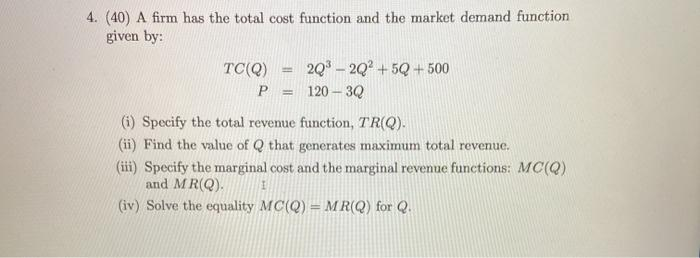### Create an Account

Home / Questions / A firm has the total cost function and the market demand function given by: TC(Q) 2Q3 – 20...

# A firm has the total cost function and the market demand function given by: TC(Q) 2Q3 – 20+5Q+500 P = 120 - 30 (i) Specify the total revenue function, TR(Q). (ii) Find the value of Q that generates

A firm has the total cost function and the market demand function given by: TC(Q) 2Q3 – 20+5Q+500 P = 120 - 30 (i) Specify the total revenue function, TR(Q). (ii) Find the value of Q that generates maximum total revenue. (iii) Specify the marginal cost and the marginal revenue functions: MC(Q) and MR(Q). (iv) Solve the equality MCQ) = MR(Q) for Q.May 10 2021 View more View LessSubscribe To Get Solution